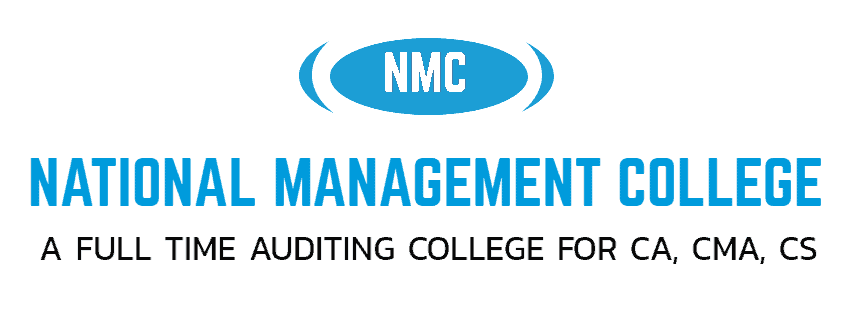# CA Foundation (Paper 3) Business Maths and Statistics 1

NATIONAL MANAGEMENT COLLEGE, THUDUPATHI.

PAPER – 3: BUSINESS MATHEMATICS, LOGICAL REASONING AND STATISTICS

Time Allowed : ½ hour                                               Maximum Marks: 25

1)If  log10 3 =  , log10 4 =  , then the value of log10 120 =

1. a) b) ) c) ) d) ) 2

2)   for some   and   , then the value of   is

1. a) 8 b) 6 c) 4 d)  2

3) . if  the ratio of two numbers is 7 : 11.  If 7 is added to each number then the new ratio will be 2 : 3 then the numbers are.

(a) 49, 77 (b) 42, 45  (c) 43,42 (d) 39, 40

4) log 2√2 (512):log3√2 (324 )  then,

(a) 128 : 81   (b) 2 : 3   (c) 3 : 2  (d) None

5)  Then  the value of

log5 + log5 + log5  + …………..log5

1. a) 2 b)3 c)5 d) none of these

6) The two numbers are in ratio 3 : 4. The difference between their squares is 28. Find the greater number

. (a) 12 (b) 8  (c) 16 (d) 10

7) In a class there are 30 boys and 12 girls. Determine the ratio of number of boys to girls.

1. a) 2 : 5 b) 3 : 6 c)  5 : 6 d)  5 : 2

8) A sum of money is to be distributed among A, B, C, D in the proportion of 5 : 2 : 4 : 3. If C gets Rs. 1000 more than D, what is B’s share?

a)Rs. 500   b) Rs. 1500  c)Rs. 2000 d)None of these

9) Find sum of all values of “a” ,

=  log 7 2401

1. a) -2 b) -12 c)-18 d) -6

10)   then all x, y, z are greater than 0 , then

1. a)b)
2. c) d)

11) . The ratio of the present age of father to that of son is 7:2. After 10 years their ages will be in the ratio of 9:4. The present ages of the father is

1. 35 years                    b. 40 years c. 30 years                                 d. 25 years

12) Price of each article of type P, Q, and R is Rs. 300, Rs. 180 and Rs. 120 respectively. Suresh buys articles of each type in the ratio 3:2:3 in Rs. 6480. How many articles of type Q did he purchase?

1. 8                       b. 14                                   c. 20                              d. None

13) .If the ratio of present ages of Jeet and Jay is 5:7 and after 6 years the ratio will be 3:4, what is the present age of Jay?

1. 42                    b. 30                           c. 36                      d. None of these

14) The triplicate ratio of 1:2 is?

1. 8:1                 b. 1:8                            c. 2:1                      d. 1:2

15) . The compound ratio of 5:6, 3:2 and 4:5?

1. 12:13           b. 1:4                             c. 1:5                     d. 1:1

16)     value

1. a) 1/6                 b)  1/2                 c) 1/3 d) 1/8

17) . log 144 =

1. a) 2 log 4 + 2 log 2 b) 4 log 2 + 2 log 3
2. c) 3 log 2 + 4 log 3 d) 3 log 2 – 4 log 3

18) For p, q, r ,s > 0  for value of each ratio is

a)1/2   b) 1/3

1. The mean proportional between 24 and 54 is
2. a) 33 b) 34 c) 35 d) 36

20) . Which of the numbers are not in proportion  ?

1. a)  6 , 8  , 5 , 7 b) 7 , 3 , 14 , 6
2. c) 18 , 27 , 12 , 18 d) 8 , 6 , 12 , 9

21)

 log0.01 (10,000) = x; Find the value of x? (a) 1(c) – 4 (b)(d) – 22

22)  What number is to added each of the ratio 7:9 to equal 15:16 ?

(a) 23 (b) 16 (c) 31 (d) 13

23) .  If  =  =  then z is equal to

1. a)  xy                         b) c) d)

24) . If  2x -2x-1 =4  then Xx =

1. a) 7                                b) 3                              c) 27                                              d) 9

25) . Find the value of x, if x (x)1/2  = (x1/2)x

a). 3                            b) 4                              c) 2                                                            d) 6